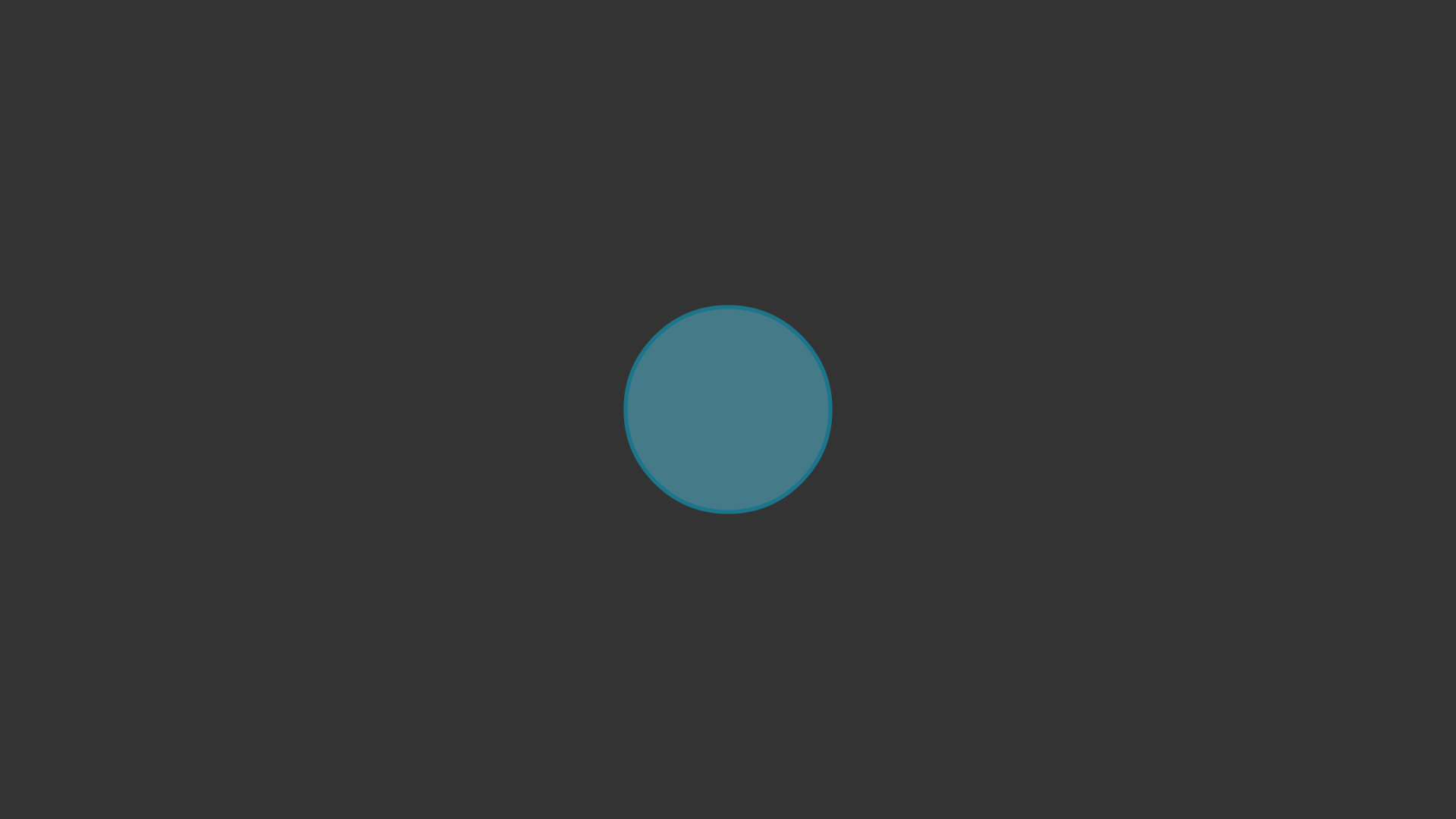# 快速入门¶

manim/
├── manimlib/
│   ├── animation/
│   ├── ...
│   ├── default_config.yml
│   └── window.py
├── (custom_config.yml)
└── start.py


 1 2 3 4 5 6 7 8 9 from manimlib import * class SquareToCircle(Scene): def construct(self): circle = Circle() circle.set_fill(BLUE, opacity=0.5) circle.set_stroke(BLUE_E, width=4) self.add(circle) 

manimgl start.py SquareToCircle


• 滚动鼠标中键来上下移动画面

• 按住键盘上 z 键的同时滚动鼠标中键来缩放画面

• 按住键盘上 f 键的同时移动鼠标来平移画面

• 按住键盘上 d 键的同时移动鼠标来改变三维视角

• 按下键盘上 r 键恢复到最初的视角

manimgl start.py SquareToCircle -os## 制作图片¶

Line 1:

from manimlib import *


Line 3:

class SquareToCircle(Scene):


Line 4:

def construct(self):


Line 5:

circle = Circle()


Line 6~7:

circle.set_fill(BLUE, opacity=0.5)
circle.set_stroke(BLUE_E, width=4)


• .set_fill() 方法将这个圆的填充颜色设为蓝色（BLUE，在 常量部分 constants 中定义），填充透明度设为0.5。

• .set_stroke() 方法将这个圆的线条颜色设为深蓝色（BLUE_E，在 常量部分 constants 中定义），线条宽度设为4

Line 9:

self.add(circle)


## 添加动画¶

  1 2 3 4 5 6 7 8 9 10 11 12 13 from manimlib import * class SquareToCircle(Scene): def construct(self): circle = Circle() circle.set_fill(BLUE, opacity=0.5) circle.set_stroke(BLUE_E, width=4) square = Square() self.play(ShowCreation(square)) self.wait() self.play(ReplacementTransform(square, circle)) self.wait() 

manimgl start.py SquareToCircle


manimgl start.py SquareToCircle -ow


Line 10:

self.play(ShowCreation(square))


Line 11:

self.wait()


Line 12:

self.play(ReplacementTransform(square, circle))


Line 13: 同Line 11，停顿1s

## 启用交互¶

self.embed()


# 在水平方向上拉伸到四倍
play(circle.animate.stretch(4, dim=0))
# 旋转90°
play(Rotate(circle, TAU / 4))
# 在向右移动2单位同时缩小为原来的1/4
play(circle.animate.shift(2 * RIGHT), circle.animate.scale(0.25))
# 为了非线性变换，给circle增加10段曲线（不会播放动画）
circle.insert_n_curves(10)
# 给circle上的所有点施加f(z)=z^2的复变换
play(circle.animate.apply_complex_function(lambda z: z**2))
# 关闭窗口并退出程序
exit()


manimgl